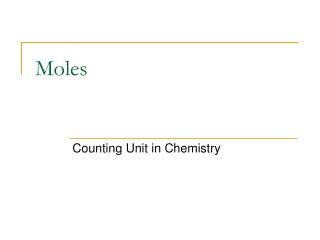DownloadDownload PresentationMoles

# Moles

Télécharger la présentation## Moles

- - - - - - - - - - - - - - - - - - - - - - - - - - - E N D - - - - - - - - - - - - - - - - - - - - - - - - - - -
##### Presentation Transcript

1. Moles Counting Unit in Chemistry

2. Agenda: • Size of Atoms • Moles: a counting unit in chemistry • What is a mole? • Why do we use moles? • Mole calculations • Factor Label Method

3. Atoms are very, very small particles • Too small to see • To small to use an optical microscope • Use a scanning tunneling microscope that is able to detect the electron clouds (due to the negative charge of the electrons)

4. Size of Atoms: DiameterSome estimates • Atoms including the electron cloud • 1 x 10⁻¹⁰ meters • Nucleus • 1 x 10⁻¹²m • Proton • 1 x 10⁻¹³ m (note: mass: 1x 672 x 10⁻²⁴g) • Electron • 1 x 10⁻¹⁶m

5. Review: Scientific Notation:aka: exponents, power of ten, etc. • Positive exponents: • 1 x 10⁶ atoms • Negative exponents: • 1 x 10⁻⁶ atoms • Multiplying with exponents: • (1.5 x 10³ atoms) x ( 4.2 x 10⁶ atoms) • (3.4 x 10³ atoms) x (2.0 x 10⁻⁶ atoms) • Dividing with exponents: • (1.5 x 10³ atoms) / ( 4.2 x 10⁶ atoms) • (3.4 x 10³ atoms) / (2.0 x 10⁻⁶ atoms) More information: Appendix near the back of the textbook

6. Moles Counting Unit in Chemistry

7. Alternative names for numbers Gross of pencils

8. Moles • Video: 4+ minutes • http://ed.ted.com/lessons/daniel-dulek-how-big-is-a-mole-not-the-animal-the-other-one • Review: • http://ed.ted.com/lessons/daniel-dulek-how-big-is-a-mole-not-the-animal-the-other-one#review • DigDeeper • http://ed.ted.com/lessons/daniel-dulek-how-big-is-a-mole-not-the-animal-the-other-one#digdeeper

9. The Mole and Avogadro’s Number • What is a mole in chemistry? • How much is a mole? • - • - • - • When are moles used?

10. Moles • Why are moles used? • How are they measured? (Standards) • The amount of substance that contains as many particles as there are in exactly 12 grams of C-12. • The amount of substance that contains Avogadro’s number of particles. • Molar mass – using the Periodic Table

11. Salt particle- NaClModel of NaCl as an ionic compound Each salt particle in this photomicrograph would require the model to be multiplied by 10⁶ or more New Term Formula Unit: NaCl Represents the repeating units that make up an ionic compound Such as NaCl, CaCl, Al₂O₃

12. Mole Conversions(Factor Label Method) • Moles ↔ Grams 3.5 moles of He = ? Grams 40 grams of He = ? Moles Molar mass: 1 mole of He = ? Grams

13. Review scientific notation Mole Conversions • Moles ↔ Particles (atoms, molecules, compounds, etc.) 3.5 moles of He = ? Atoms 12.04 x 10²³ atoms of He = ? Moles 1 mole = atoms

14. Mole Conversions • Moles ↔ Volume of Gas (at STP) 3.5 moles of He = ? Liters 100 Liters of He = ? Moles 1 mole of a gas = Liters

15. Mole Flow Chart Mole

16. Practice problems: Moles and Mass • What is the mass of 0.5 moles of S? • How many grams is equivalent to 0.137 moles of Fe? • 4.63 mol P = ? grams P

17. Practice problems • How many moles are in 84.3 grams of Si? • 50 grams of Ne = ? moles of Ne

18. Mole and Atom Conversions • How many atoms of Al are in 2.5 moles? • How many atoms of Ag are in 0.26 moles? • How many atoms of He are in 0.0023 moles?

19. Mole and Atom Conversion • How many moles of P contains 1.0 x 10²³ atoms? • How many moles of Au are in 2.8 x 10²º atoms?

20. Moles, Moles, Moles Lab Sample in a jar Mass Moles Atoms 1 mole = ? grams 1 mole = ? atoms

21. Molar Mass of Compounds • NaOH H₃PO₄ • H₂O PCl₃ • MgCl₂ H₂SO₄

22. Mole Conversion of Compounds • How many grams are in 5 moles of CO₂? • How many atoms are in 5 moles of CO₂? • How many liters are in 5 moles of CO₂?

23. A Mole is a Number

24. Review

25. Carbon has 6 protons